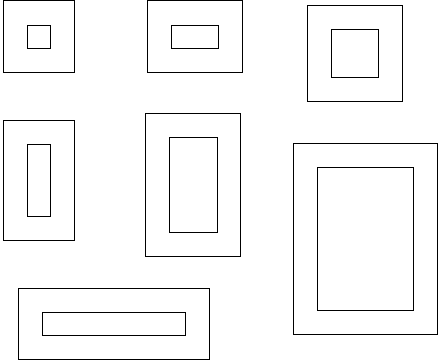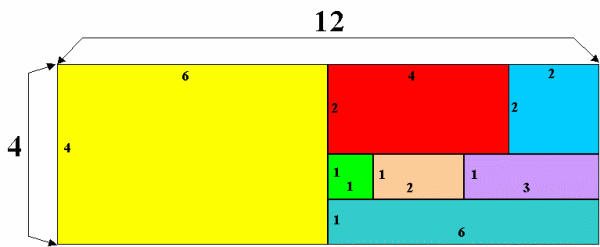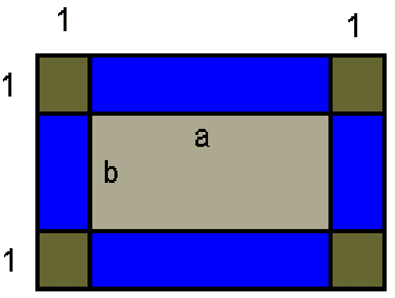#### You may also like### All in the Mind

Imagine you are suspending a cube from one vertex and allowing it to hang freely. What shape does the surface of the water make around the cube?### Instant Insanity

Given the nets of 4 cubes with the faces coloured in 4 colours, build a tower so that on each vertical wall no colour is repeated, that is all 4 colours appear.### Is There a Theorem?

Draw a square. A second square of the same size slides around the first always maintaining contact and keeping the same orientation. How far does the dot travel?

# Framed

##### Age 11 to 14Challenge Level

Well done to Prateek Mehrotra (aged 14) from Riccarton High School in Christchurch, New Zealand, Jenny Anderton (aged 15) from The Mount School in York and Andrei Lazanu (aged 12) from School No. 205 in Bucharest, Romania who have all sent us the correct solution.

The pictures are1$\times$1, 1$\times$2, 2$\times$2, 1$\times$3, 2$\times$4, 4$\times$6 and 1$\times$6.The areas of the pictures add up to 48 so they fit into a 4$\times$12 rectangle.

This is how they fit :This is how Andrei Lazanu worked out the solution:

Let $a$ and $b$ be the sides of the rectangular picture and the area of the frame is :
$(a+2)(b+2)-ab = ab + 2a + 2b + 4 -ab = 2a + 2b + 4$
as represented in the picture below:$2a + 2b + 4 = xab$, where $x$ is the number of times the area of the frame enters in the area of the rectangle. $a$, $b$ and $x$ are positive integers.

I start with $x = 1$.

$2a + 2b + 4 = ab$. I subtract $2b$
$2a + 4 = b(a - 2)$. I calculate $b$: $${b} = { {2a+4}\over{a-2}} = {{2(a+2)}\over{a-2}} = {{2(a-2+4)}\over{a-2}} = {{{2(a-2)}\over{a-2}} + {8\over{a-2}}} = { {2} + {8\over{a-2}}}$$

So, 8 must be divisible by $(a-2)$. In this case $(a-2)$ can be 8, 4, 2 or 1 and a can be 10, 6, 4 and 3 respectively.

 If $a$ is 10, $b$ is 3 and the area is 30. (1) If $a$ is 6, $b$ is 4 and the area is 24. (2) If $a$ is 4, $b$ is 6 and the area is 24. (3) If $a$ is 3, $b$ is 10 and the area is 30. (4)

(1) is equivalent with (4) and (2) with (3), because they have the same area, and the sides of the rectangle are reversed.

I analyse $x=2$.

$2a + 2b + 4 = 2ab$. I subtract $2b$.
$2a + 4 = 2b(a - 1)$.
$2(a + 2) = 2b(a - 1)$. Divide both members by 2.
$a + 2 = b(a - 1)$. I calculate $b$. $${b} = { {a+2}\over{a-1}} = {{a - 1 + 3}\over{a-1}} = {{(a - 1) +3}\over {a - 1}}= { {1} + {3\over{a-1}}}$$So, 3 must be divisible by $(a - 1)$. In this case $(a - 1)$ can be 3 or 1, so a can be 4 or 2 respectively.

 If $a$ is 4, $b$ is 2 and the area is 8. (5) If $a$ is 2, $b$ is 4 and the area is 8. (6)

(5) is equivalent with (6).

I analyse $x=3$.

$2a + 2b + 4 = 3ab$. I subtract $2b$.
$2a + 4 = b(3a - 2)$. I calculate $b$. $${b} = { {2a+4}\over{3a-2}} = {{2(a + 2)}\over{3a-2}}$$

Because this expression is difficult to write in other terms, to analyse divisibility criteria, I try different values for $a$, to see when I can obtain b integer.

• $a = 1$. ${b} = {{2\times 3}\over1} = {6}$ For $a = 1$, $b = 6$.
• $a = 2$. ${b} = {{2\times 4}\over4} = {2}$For $a = 2$, $b = 2$.
• $a = 3$. ${b} = {{2\times 5}\over7}$ $b$ isn't an integer.
• $a = 4$. ${b} = {{2\times 6}\over10}$ $b$ isn't an integer.
• $a = 5$. ${b} = {{2\times 7}\over13}$ $b$ isn't an integer
• $a = 6$. ${b} = {{2\times 8}\over16} = {1}$For $a$ = 6, $b$ = 1.

Now I don't try higher values for $a$, because $b$ will be less than 1, and therefore not an integer.

I analyse $x = 4$.

$2a + 2b + 4 = 4ab$.
$2(a + b + 2)= 4ab$. I divide both members by 2.
$a + b + 2 = 2ab$. I subtract $b$ $${b} = {{a + 2}\over{2a - 1}}$$

• I try a = 1. ${b} = {{3}\over{1}}$For $a = 1$, $b = 3$
• I try $a = 2$. ${b} = {{4}\over{3}}$ $b$ isn't an integer.
• I try $a = 3$. ${b} = {{5}\over{5}}$For $a = 3$, $b = 1$.

a cannot be greater than 3 in order to have $b$ integer.

I analyse $x = 5$.

$2a + 2b + 4 = 5ab$. I subtract $2b$.
$2a + 4 = b(5a - 2)$. $${b} = {{2(a + 2)}\over {5a + 2}}$$

• I try $a = 1$. ${b} = {{6}\over{3}} = {2}$ For $a = 1$, $b = 2$.
• I try $a = 2$. ${b} = {{8}\over{8}} = {1}$ For $a = 2$, $b = 1$.
Which is the same as before with $a$ and $b$ interchanged.

I analyse $x = 6$.

$2a + 2b + 4 = 6ab$
$2(a + b + 2) = 6ab$. I divide both members by 2.
$a + b + 2 = 3ab$. I subtract $b$.
$a + 2 = b(3a - 1)$ $${b} = {{a + 2}\over {3a - 1}}$$

• I try $a = 1$. $${b} = {{3}\over{2}}, b \mbox{isnt an integer}$$.

• I try $a=2$. $${b} = {{4}\over{5}}, b \mbox{isnt an integer}$$.

I analyse $x=7$.

$2a + 2b + 4 = 7ab$. I subtract $2b$.
$2a + 4 = b(7a - 2)$ $${b} = {{2a + 4}\over {7a - 2}}$$

• I try $a = 1$. ${b} = {{6}\over{5}}, b \mbox{isnt an integer}$.
• I try $a = 2$. ${b} = {{8}\over{10}}, b \mbox{isnt an integer}$.

I analyse $x=8$ .

$2a + 2b + 4 = 8ab$
$2(a + b + 2) = 8ab$. I divide both members by 2.
$a + b + 2 = 4ab$. I subtract $b$.
$a + 2 = b(4a - 1)$. $${b} = {{a + 2}\over {4a - 1}}$$

Try $a$ = 1. $${b} = {{3}\over{3}}= {1}$$

For $a$ = 1, $b$ = 1.

Now, I don't look for $x$ greater than 8, because I obtained the smallest sides possible.

These are all the numbers I found. They are written in the following table:

a b ab
4 6 24
3 10 30
2 4 8
1 6 6
2 2 4
1 3 3
1 2 2
1 1 1

There are 8 sets of numbers and the sum of their areas is:

24 + 30 + 8 + 6 + 4 + 3 + 2 + 1 = 78 (in 2 )

But I must obtain for this sum a multiple of 12 using 7 sets of numbers.
The multiples of 12 below 78 that I can obtain are:

 72 = 78 - 6 60 = 78 - 18 (but there isn't an 18 in the list of the areas) 48 = 78 - 30

So, I can obtain only 72 (12$\times$6) and 48 (12$\times$4).

But using 30 and 24, it isn't possible to create a rectangle with sides 12 and 6 inches, because there is no way to arrange them.

So, I can only create a pattern of 48 in 2 , with the other side 4 inches long.

In conclusion, this problem has a unique solution.